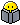# full mesh formula

Member Posts: 130 ■■■□□□□□□□
Hi guy/gals

We all know the formula of full mesh topology w=n*(n-1)/2 w= number of links n=number of sites. Let say I have 8 sites I need to find the number of links. According to this formula 8*(8-1)/2. So 8-1 =7/2 = 3.5*8=24.5 In such case we do have round to 24 or 25?

Thank you all for your input
working on CCNA

• Member Posts: 29 ■□□□□□□□□□
PEMDAS

8*(8-1)/2 does not = 24.5

Parenthesis = 8-1=7
Exponents = N/A
Multiplication = 8*7=56
Division = 56/2=28
Got me too the first time I tried your example.